Forgot your password? User-login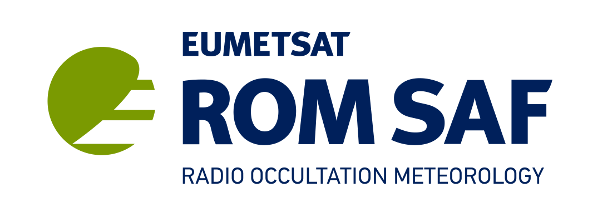|||
 About Home RO method News Archive Contact Search Documentation Product Documents Publications ROM SAF Reports Visiting Scientist User Workshops Data & Software Product Archive Product Quality Browse Occultations NRT Monitoring Climate Monitoring Software User Service Helpdesk Helpdesk History RSS Feeds Registration Login
 NWP Model and Data Flow Matched Occultations Matched Radiosondes Monitoring Description

# Old Monitoring: Description

This page contains the NRT monitoring of data up to 1st May 2017. Improved NRT monitoring of RO data can be found at NRT monitoring starting at 1st May 2017.

## Definitions, acronyms and abbreviations:

B Forward modelled refractivity or bending angle (referred to as background)
BUFR Binary Universal Form for the Representation of data
CDOP Continuous Development and Operational Phase (SAFs)
COSMIC Constellation Observing System for Meteorology, Ionosphere and Climate
DMI Danish Meteorological Institute
ECMWF European Centre for Medium-Range Weather Forecasts
EUMETSAT European Organisation for the Exploitation of Meteorological Satellites
GFZ German Research Centre for Geosciences
GNSS Global Navigation Satellite Systems (generic name for GPS, GLONASS and the future GALILEO)
GPS Global Positioning System (US)
GRAS GNSS Receiver for Atmospheric Sounding (on-board Metop)
GRAS SAF Previous name for the ROM SAF
GTS Global Telecommunications System (WMO)
MetDB The Met Office's database for observations
Metop Meteorological Operational polar satellites (EUMETSAT)
NRT Near Real Time
NWP Numerical Weather Prediction
O Observed refractivity or bending angle
Operational ROM SAF Team responsible for the handling of GRAS data and the delivery of meteorological products during the operational life of the instrument
POD Precise Orbit Determination
PPF Product Processing Facility
QC Quality Control
ROM SAF (formerly known as GRAS SAF) Radio Occultation Meteorology SAF. Consortium formed to define and prepare the Operational ROM SAF. Members are DMI(leader), Met Office, ECMWF and IEEC.
SAC-CSatelite de Aplicaciones Cientificas-C
SAF Satellite Application Facility (EUMETSAT)
UCAR University Corporation for Atmospheric Research
UTC Universal Time Coordinated
VAR Variational analysis; 1D, 2D, 3D or 4D versions (NWP data assimilation technique)
WMO World Meteorological Organisation

## The data:

The monitored radio occultation data are provided to the Met Office as refractivity and bending angle profiles processed by the ROM SAF (for the GRAS instrument), GFZ (CHAMP and GRACE-A satellites) and from UCAR (FORMOSAT-3/COSMIC, SAC-C and C/NOFS). The GRAS observation data used in the plots are obtained from DMI BUFR files, which are disseminated in NRT via the GTS. Any GRAS plots specified as ECMWF data will be using only bending angle data, which is sourced from EUMETSAT BUFR files (the ECMWF statistics are calculated at ECMWF and received at the Met Office already processed to (O-B) statistics). Most plots are based on the comparison of observation data to forward modelled Met Office global model (operational) 6 hour forecast data and forward modelled ECMWF (operational) 12 hour forecast data (upgrades to these NWP systems are logged here). The refractivity and bending angle forward models are currently both 1D local operators (see ROPP documentation for bending angle operator description).

The Met Office refractivity forward model simulates refractivity as a function of geopotential height and is relatively straightforward. It requires model total pressure and specific humidity on geopotential heights horizontally interpolated to observation location, from which temperature can be calculated and hence the refractivity (using an empirical formula). This is followed by a vertical interpolation to the observation heights. The bending angle operator follows the same method up to producing refractivity on model levels, the bending angle is then calculated using the inverse Abel transform (as in ROPP). The ECMWF data use a very similar bending angle forward model to the one employed by the Met Office.

The IDL monitoring is automated so that the plots on the web site are updated every 6 hours with a delay of roughly 8 hours since the last model run, and is based on roughly the previous 30 days data. Note that this system is not operational and there may be short periods when the plots do not get updated. The majority of the monitoring plots are based on (O-B)/B statistics (using the Met Office global model 6 hour forecasts as B unless otherwise stated). The plots should represent the quality of the data we receive at the Met Office (and therefore any other NWP centre) taking into account the idiosyncrasies of the model (unique biases). However, it should be noted that some quality control is performed to remove extreme outliers. Firstly we disregard any unphysical data in the observation and background e.g. refractivity, bending angles and associated height co-ordinates less than zero. We then do a check for extreme outliers in the (O-B)/B on a level-by-level basis: we reject any values with |(O-B)/B| > 10*ob_error% where ob_error is the observation plus forward model assumed errors. For bending angle the assumed errors are the same as those used at ECMWF.

## Data Quality Monitoring:

(O-B)/B profile plots
These plots allow us to compare the refractivity (or bending angle) calculated using the Met Office's global model fields with the refractivity (or bending angle) data given in a corresponding GPSRO observation. The fractional difference in refractivity (or bending angle): (O-B)/B, is calculated for each occultation profile at a fixed set of altitude levels (200 m intervals, the data were interpolated on to these levels using a log-linear method). The altitude data are geopotential height with respect to the geoid for refractivity and impact height with respect to WGS-84 ellipsoid for bending angle.

The mean of (O-B)/B over many occultations for each altitude level is calculated. Over many thousands of occultations this mean indicates the typical differences (biases) between the observed and modelled refractivity (or bending angle). We also show the standard deviation of (O-B)/B, indicating the width of the distribution. The plots show the number of occultations used in each mean and standard deviation calculation i.e. number that got past the QC.

The (O-B)/B plots are separated into: global data, latitudinal bands; northern/southern hemispheres/tropics, land/sea, setting/rising and nominal/no-nominal. Bending angle (O-B)/B statistics provided by ECMWF have been added to the global and northern/southern hemispheres/tropics (O-B)/B plots. The profile plots are useful for comparing satellites/models/processing centres and thus for making decisions on which data to assimilate into NWP models. The land/sea bias plots were created using a land-sea mask on a resolution of 1 degree in latitude and longitude.

top

Time-trend plots
These plots show how global refractivity (O-B)/B statistics vary with time on altitude levels. The mean (O-B)/B over a 6-hour period is plotted as a the colour of a box for a given time and altitude, this is repeated for all times and altitudes for the data over the defined period. Similarly plots of standard deviation and a count of the number of occultations used in the mean and standard deviation calculations are produced. The plots allow changes in the data quality of the observations or background to be seen. Also available are ECMWF and Met Office bending angle statistics against time on the same plot for a selection of impact heights; with Met Office statistics plotted every 6 hours, ECMWF every 12 hours. They help to determine whether changes with time are related to observations or the models.

Zonal (O-B)/B
These plots show the refractivity (and bending angle) (O-B)/B mean, (O-B)/B standard deviation and observation count averaged over latitudinal bands around the globe. Each band is 4 degrees wide in latitude to ensure sufficient data for the average. The zonal averages are calculated on each altitude level, and the result is shown as a contour plot.

(O-B)/B histogram plots
Histograms of (O-B)/B values are produced for all the altitude levels for refractivity and bending angle. The histograms are normalised (so the area under the histogram at any particular height level is 1), hence the axis label PDF (probability density function, a reasonable estimate given a large enough sample). Given a histogram for each level we then plot each one against altitude with the value of the PDF shown as a colour. The plot gives a lot more information on the spread of the (O-B)/B compared to simply the standard deviation i.e. the shape of the distribution with altitude can be seen.

1D-Var stats
At the Met Office we pass radio occultation data through a 1D-Var procedure for QC purposes prior to assimilating the data in 4D-Var; the aim of this is to black-list poor quality occultations. We run this 1D-Var in a non-operational capacity for monitoring. We set the 1D-Var to have no vertical cut-off (typically 0 to 60 km) and to use all the data received at the Met Office i.e. no QC prior to 1D-Var is done.

The 1D-Var hist plot shows histograms of the number of iterations required to converge, 2J/m (where J =cost function at solution and m = number of observations in a profile), 2J/m when J is the initial cost function and DFS (degrees of freedom of signal) which is calculated as the trace of AKTO-1K where A is the solution error covariance matrix, K is the forward model Jacobian matrix and O is the observation plus forward model error covariance matrix (see Inverse Methods for Atmospheric Sounding C.D. Rodgers pages 33,37).

The 1D-Var time series plot shows the trend of the 1D-Var statistics: the number rejected (and why) and the 2J/m value on first and final iterations. 2J/m should be roughly 1 if the background and observation plus forward model errors are specified correctly. Another plot shows 2J/m vs latitude, i.e. the final 2J/m against tangent point latitude of the occultation. Both plots are useful for determining the quality of data.

Occultation map
The plot shows the position on a global map of the occultations for the chosen satellite over the designated time period. The colour of the marker for the occultation position corresponds to the lowest geopotential height provided with the refractivity profile. The height can be read from the colour bar provided below the map. The shape of the marker indicates whether it is a rising or setting occultation. The plot provides an indication of the global spread of the data and allows one to see any spatial patterns in lowest processed height. Another map (Gradient map) plot shows the altitude at which the observation and model background gradient of refractivity is less than -100 N/km shown at the locations of the occultations. This indicates where on the globe and a what heights super-refraction is likely.

top

Correlation/Covariance plots
To get a qualitative feel for observation error covariance, the vertical correlation and vertical covariance matrices of (O-B)/B for refractivity (bending angle) plotted against geopotential height (impact height) are provided. Although clearly some of the correlation of (O-B)/B comes from B (the model refractivity or bending angle) we can still get a feel for the observation part of error correlation by performing comparisons between satellites/processing centres, when B should be the same (although different satellites sample different local times). Also the difference between refractivity and bending angle provide an interesting comparison.

Matched occultation plots
Given an occultation one can search for other occultations that lie within a specified time-range and distance from the reference occultation. Statistical comparisons of the refractivity/bending angle observation data from these matches can then be made. The plots show how the mean and standard deviation of the fractional difference vary with latitude between different GPSRO receivers and processing centre combinations. Currently the criteria for a match is that the great-circle distance is less than 300 km and the time difference is less than 3 hours. Surrounding the mean in a dashed line is the 95% confidence interval on the mean to help judge the significance of the result.

Given an occultation one can search for radiosondes that lie within a specified time-range and distance from the reference occultation. Statistical comparisons of the refractivity GPSRO observation data to the radiosonde-derived refractivity can then be made. The plots show how the mean and standard deviation of the fractional difference vary with latitude between different GPSRO receivers and radiosonde type combinations. Currently the criteria for a match is that the great-circle distance is less than 300 km and the time difference is less than 3 hours. Surrounding the mean in a dashed line is the 95% confidence interval on the mean to help judge the significance of the result. The plots are also split by day/night-time to help distinguish the effects of diurnal radiosonde biases. For the calculation of radiosonde refractivity we use the commonly used Smith & Weintraub formula, given the radiosonde temperature and dew-point temperature as a function of pressure. The geopotential heights are calculated by summing thicknesses between radiosonde significant level data using the hypsometric equation. The calculated radiosonde refractivity is interpolated onto the standard set of GPSRO geopotential heights used in the other plots using the same log-linear method.

## Data Flow Monitoring:

These plots show statistics of the time delay between the observation time and the time of receipt at the Met Office.

Delay histograms
• Plots are for individual satellite/processing centre combos
• The title shows the satellite and processing centre IDs and the first and last observation times being analysed. The period will either be for one day ('yesterday') or a nominal 28 days ('month')
• Dotted lines represent the operational target that at least 90% of the data should arrive within 3h00m of observation time
• Solid bars are the histograms of delays. coloured according to the delay thresholds:
• Green - under 3 hours
• Yellow - 3 to 6 hours
• Red - over 6 hours
• Bins are counts over 30-minute intervals and the last bin is the count of all delays of more than 12 hours
• Dashed lines are accumulation histograms (1-minute resolution), which can be compared to the targets
• If there were no data for a satellite/centre in the period being analysed, the plot will be blank
• The date and time when the plots were generated are shown in the bottom right corner - if not today, this will indicate if the plots are not being updated correctly.
Summary statistical data is also shown on each plot:
• # Samples: number of GPSRO profiles received with observation times falling within the period shown in the plot title
• Minimum: the shortest delay
• Average: the average (mean) delay
• Maximum: the longest delay
• 50% in and 90% in: the delay for which the target accumulated amount of data was available
• Obs <3h00m: the number of profiles available within the target delay time as a percentage of all those received for the period.

These plots are produced for yesterday's data and for a 28-day (monthly) period of data.They are important for judging how quickly the data is (or could be) assimilated into NWP models.

top

Data availability
• Plots are for individual satellite/processing centre combos
• The title shows the satellite and processing centre IDs and first and last observation times being analysed; nominally this will be the previous 28 days
• The vertical axis denotes the date (dd/mm) and the horizontal axis the UTC time of day (hh) of the observation
• A coloured box is plotted for an hour & date if at least one RO profile was available in that hour; the number of profiles received is printed within the box.
• The colour of the box indicates the smallest delay for data observed within that hour
• Green - under 3 hours
• Yellow - 3 to 6 hours
• Red - over 6 hours
• The key at the bottom of the plot shows the colours used. Blank areas mean that no profiles were available in MetDB for that hour (at the time of the analysis)
• The total number of profiles for each day is shown on the right margin of the plot and the overall total at the bottom of the column.
• The date and time when the plots were generated are shown in the bottom right corner - if not today, this will indicate if the plots are not being updated correctly.

It should be noted that the flow of data provided to the Met Office can stop occasionally for reasons beyond our control, hence why some of the plots may be blank.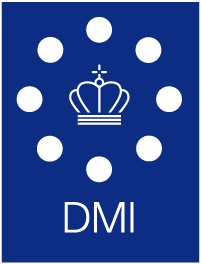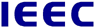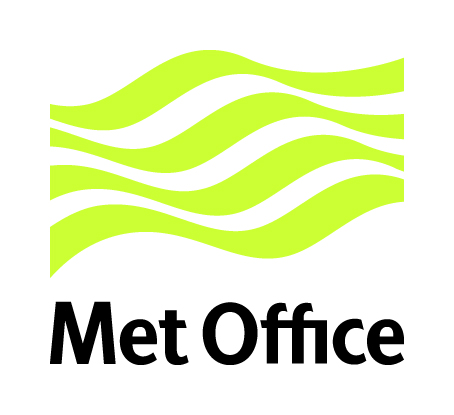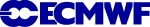Copyright © 1999-2023 EUMETSAT